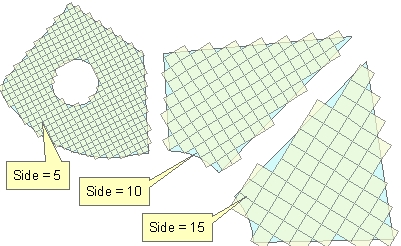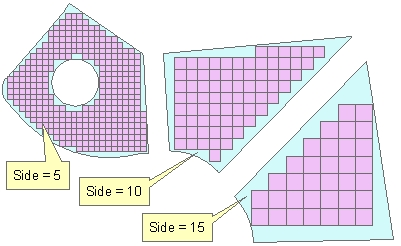Go to ET GeoWizards 12.x User Guide

Square Grids In Polygons

Go to ToolBox Implementation

Generates square grids located in the polygons of the input polygon dataset. The cell size for each polygon can be the same or different - based on the values in a numeric field of the input polygon feature class. The user can specify the rotation angle for the resulting square grids.

Inputs:

• A polygon feature class
• The cell size of the grid for each polygon can be input in one of the following ways
• A numeric field which values will define the cell size for the grid for each polygon.
• A constant number that defines the cell size (the same for all polygons)
• Rotation angle
• Constant for all polygons - (in degrees starting from East anti-clockwise)
• From field - different rotation angle for each field (in degrees starting from East anti-clockwise)
• Along the longest axis of each polygon
• Along the longest side of each polygon
• Squares completely inside the polygons
• if TRUE - no square will intersect the polygon boundary.
• If FALSE - the centers of the squares will be inside the polygons, but the squares might intersect the polygon boundary

Outputs:

• New Polygon feature class.
• The following fields are added to the attribute table of the resulting feature class.
• [ET_ID] - the ID of the original polygon

Illustration:

 Cell size = 10, Rotational angle = "Along the longest side"Completely inside = TRUEOriginal polygons labeled with the values in field to be used as a source for the Cell Size.Rotational angle = "Along the longest Axis"Completely inside = FALSEOriginal polygons labeled with the values in field to be used as a source for the Cell Size.Rotational angle = 0 Completely inside = TRUECommand line syntax

ET_GPSquareGridsInPolygons<input_dataset>  <out_feature_class> {side_length} {side_length_field} {angle_from} {rotation_angle} {angle_field} {inside_only}

Parameters

Expression Explanation
<input_dataset> A Polygon feature class or feature layer
<out_feature_class> A String - the full name of the output feature class.
{side_length} A Double representing the cell size for all polygons
{side_length_field}  A String representing the name of a numeric field which values will be used to get the cell size for each polygon
{angle_from} A String defining how the rotation angle will be specified
• "Constant" - Constant for all polygons - (in degrees starting from East anti-clockwise)
• "From field" - different rotation angle for each field (in degrees starting from East anti-clockwise)
• "Longest Axis" - Along the longest axis of each polygon
• "Longest Side" - Along the longest side of each polygon
{rotation_angle} A Double - specifying the rotation angle for all polygons.
{angle_field} A String - the name of the field to be used as source for rotation angle.
{inside_only} A Boolean defining whether the resulting square can intersect the polygon boundary or not.

Scripting syntax

ET_GPSquareGridsInPolygons(input_dataset,  out_feature_class, side_length, side_length_field,  angle_from, rotation_angle, angle_field, inside_only)

See the explanations above:
<> - required parameter
{} - optional parameter